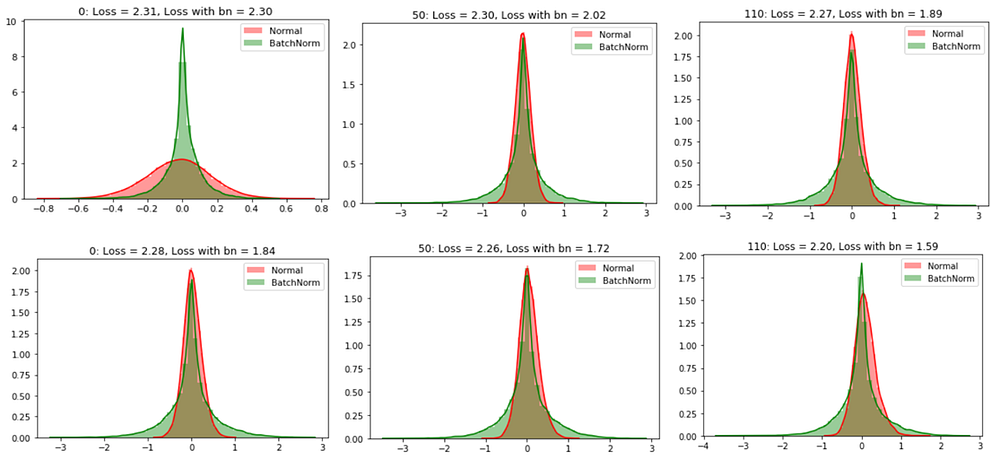# Batch Normalization and Dropout in Neural Networks Explained with Pytorch

Source: Deep Learning on MediumIn this article, we will discuss why we need batch normalization and dropout in deep neural networks followed by experiments using Pytorch on a standard data set to see the effects of batch normalization and dropout. This article is based on my understanding of deep learning lectures from PadhAI.

# Why Normalize Inputs?

Before we discuss batch normalization, we will learn about why normalizing the inputs speed up the training of a neural network.

Consider a scenario where we have 2D data with features x_1 and x_2 going into a neural network. One of these features x_1 has a wider spread from -200 to 200 and another feature x_2 has a narrower spread from -10 to 10.

Once we normalized the data, the spread of the data for both the features is concentrated in one region ie… from -2 to 2. Spread would look like this,

Let’s discuss why normalizing inputs help?

Before we normalized the inputs, the weights associated with these inputs would vary a lot because the input features present in different ranges varying from -200 to 200 and from -2 to 2. To accommodate this range difference between the features some weights would have to be large and then some have to be small. If we have larger weights then the updates associated with the back-propagation would also be large and vice versa. Because of this uneven distribution of weights for the inputs, the learning algorithm keeps oscillating in the plateau region before it finds the global minima.

To avoid the learning algorithm spend much time oscillating in the plateau, we normalize the input features such that all the features would be on the same scale. Since our inputs are on the same scale, the weights associated with them would also be on the same scale. Thus helping the network to train faster.

# Batch Normalization

We have normalized the inputs but what about hidden representatives?

By normalizing the inputs we are able to bring all the inputs features to the same scale. In the neural network, we need to compute the pre-activation for the first neuron of the first layer a₁₁. We know that pre-activation is nothing but the weighted sum of inputs plus bias. In other words, it is the dot product between the first row of the weight matrix W₁ and the input matrix X plus bias b₁₁.